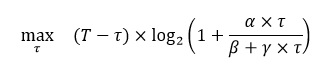# Optimizing (1-x) log(1+(g(x)/h(x)))

I want to solve the following optimization problem:

\eqalign{\max_x\quad & (1-x) \log_2 \left(1+ \underbrace{\left(\frac{xD - (1-x)E}{(1-x)F+(1-x)G}\right)}_A\right)}

Such that P_1<x\leq P_2 where, D, E, F, G, P_1, and P_2 are positive real numbers.

My attempted solution:

Since at higher values of A it is also possible to approximate the logarithmic function to

(1-x) \log_2 \left(1+ \left(\frac{xD - (1-x)E}{(1-x)F+(1-x)G}\right)\right) \approx (1-x) \log_2 \left(\frac{xD - (1-x)E}{(1-x)F+(1-x)G}\right)

I tried to solve the inner part, i.e., \left(A=\frac{xD - (1-x)E}{(1-x)F+(1-x)G}\right) using fractional programing (such as Dinkelbach Algorithm), but I am not sure how to tackle this problem as a whole in CVX.

Any kind of help in this regard will be very much appreciated.

I think you can use rel_entr, as shown in the last post in Writing a constraint in DCP complient format .

I think that would be (you better check that this is correct)

cvx_begin
variable x
maximize(-rel_entr(1-x,1-x+(x*D-(1-x)*E)/(F+G))/log(2))
P1 <= x <= P2
cvx_end


If x needs to be strictly greater than P1, then use P1 + a small number, such as 1e-6.

2 Likes

Thanks @Mark_L_Stone. I have a similar problem with a small change where instead of only (1-x) there is another constant c in multiples of (1-x). Can I write the expression as maximize(-rel\_entr(c*(1-x), c*(1-x)+(c*(1-x)*D-(1-x)*E)/(F+G))/\log(2)).
Also, if my equation is a little bit different I cannot use (rel_entr), that equation is
c*(1-x)* (1-b) * \log_2\left(\frac {(1-x)*D-(1-x)*E}{(1-x)*F + G}\right).
which is concave for the values of x between 0 and 1.

Ohh Mark, Thank you so much. I owe you a coffee, let me know if I can buy you a starbuck somehow@Danny_Wiji I’m not clear on what your first (new) expression is supposed to be. If c in an extra multiplicative factor outside of log, you can just multiply the rel_entr by it (on the outside). If it appears in some other manner, I don’t know in what manner that is.

Your second “equation” matches the definition of rel_entr once once you move around some constant factors. I leave it to you to check that the following is correct:
c*(1-b)*rel_entr(1-x,((1-x)*P+G)/(D+E))/log(2)

Note that if c > 0 and b < 1, then this expression is convex, not concave.

P.S. It seems a little strange that there are 2 such similar questions at the same time. If this is homework, please ask the professor to give ma an A (also the creator of this “trick”, mcg).

@Mark_L_Stone thanks again for your help. It clears up many things. The rel_entr form is right which you have written and yes that is convex.

c*(1-x)* (1-b) * \log_2\left(\frac {x*D-(1-x)*E}{((1-x)*F) + G}\right).
I find this expression concave when I plot it for the values of x from 0 to 1 in Matlab, where c > 0 and b < 1.
Note that this expression is a bit different from the previous one.

P.S.: LOL. You and mcg already got an A from me because you are helping me a lot.
And this is not a homework I am just trying to understand how to translate log functions into rel_entr.

Your most recent expression is not necessarily convex or concave, perhaps depending on the values of the constants. For instance, if c > 0, b < 1, D = 2, E = 1, F = 1, and G = 1, then the 2nd derivative is negative for x = 0.7 and positive for x = 0.8. So your only hope of having this be concave or convex, and potentially accepted by CVX, depends on what the values of those constants are.

I think by this point, you should have the idea of how to use the properties of log to manipulate expressions into a form which can be accepted by CVX, presuming there is such a form.

Thank you very much!! I think I owe you a coffe, too.

I don’t see how you would get that error message unless you are using gp mode.

Presuming you are not using gp mode, the following should work:

cvx_begin
variable t
h = (beta + gama*t)/alpha;
maximize((-rel_entr(h+t,h)-rel_entr(h,h+t))/log(2))My immediately preceding post addressed the -t*log2(...) term., which answered a post you subsequently deleted.
The T*log2(...) term is also concave, and i think can be handled by adapting the solution at Can CVX solve this kind of function {x-log(1+0.01*x/(x+1))} . I leave the details of the adapting to you.